# ISEE Middle Level Quantitative : Geometry

## Example Questions

1 2 19 20 21 22 23 24 25 27 Next →

### Example Question #651 : Isee Middle Level (Grades 7 8) Quantitative Reasoning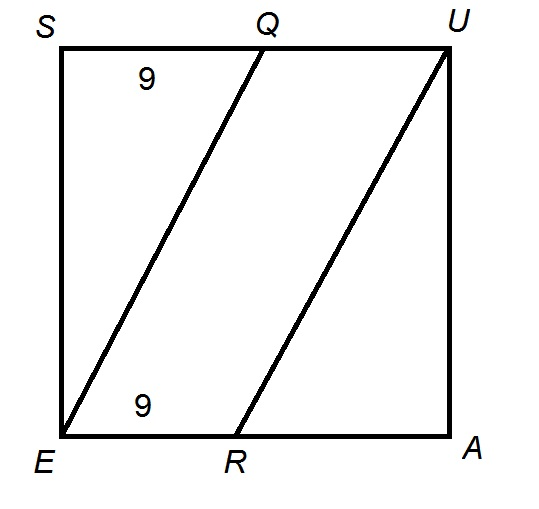Figure NOT drawn to scale.

In the above diagram, Square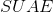has area 400. Which is the greater quantity?

(a) The area of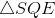(b) The area of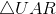(a) and (b) are equal

It is impossible to determine which is greater from the information given

(b) is the greater quantity

(a) is the greater quantity

(b) is the greater quantity

Explanation:

Squarehas area 400, so its common sidelength is the square root of 400, or 20. Therefore,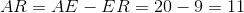.

The area of a right triangle is half the product of the lengths of its legs.has legs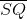and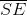, so its area is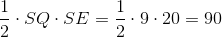.has legs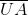and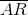, so its area is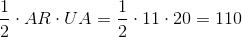.has the greater area.

### Example Question #651 : Isee Middle Level (Grades 7 8) Quantitative Reasoning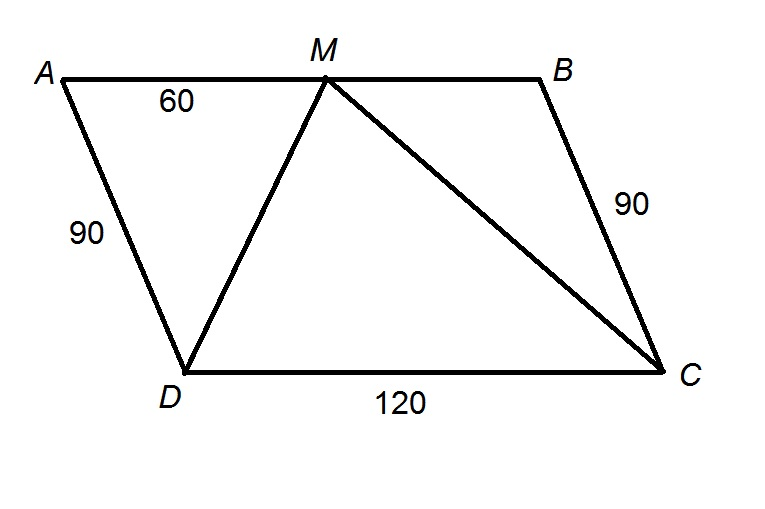Figure NOT drawn to scale

The above diagram depicts Parallelogram. Which is the greater quantity?

(a) The area of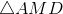(b) The area of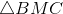(a) and (b) are equal

It is impossible to determine which is greater from the information given

(a) is the greater quantity

(a) and (b) are equal

Explanation:

Opposite sides of a parallelogram have the same measure, so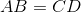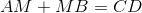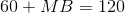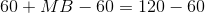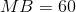Base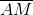ofand base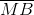ofhave the same length;  also, as can be seen below, both have the same height, which is the height of the parallelogram.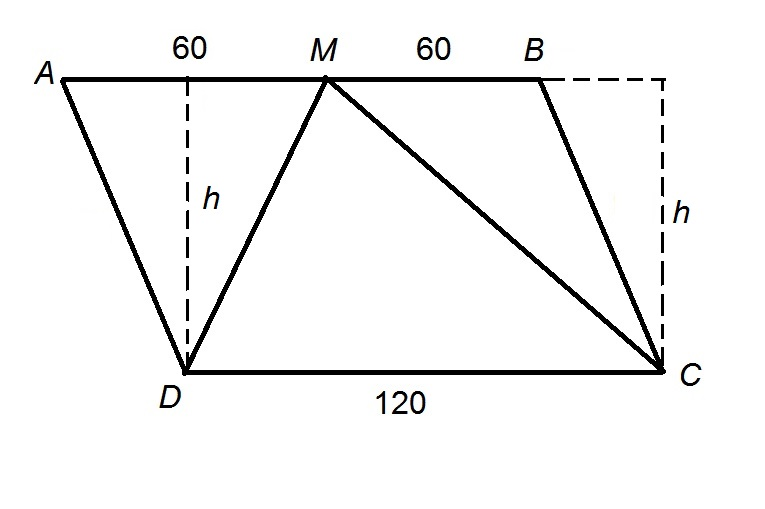Therefore, the areas ofandhave the same area -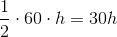.

### Example Question #2 : How To Find The Area Of A Triangle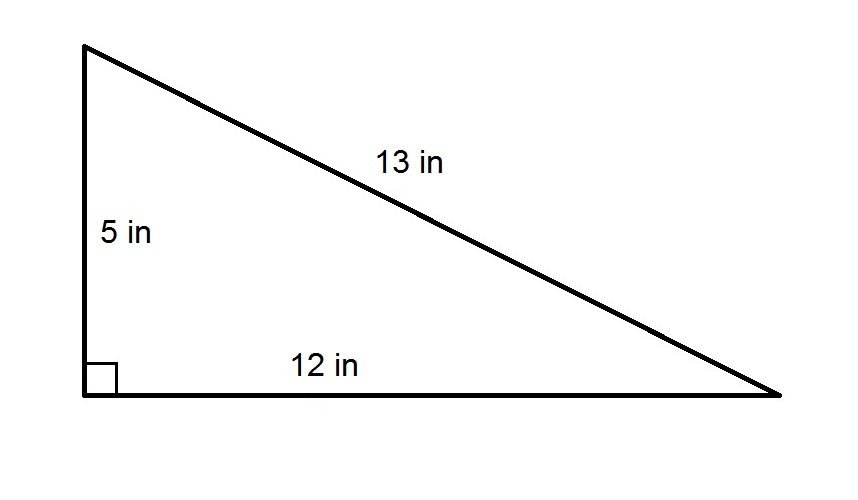Refer to the above figure. Which is the greater quantity?

(a) The perimeter of the triangle

(b) 3 feet

(a) and (b) are equal

(a) is the greater quantity

(b) is the greater quantity

It is impossible to determine which quantity is the greater from the information given

(b) is the greater quantity

Explanation:

The perimeter of the triangle - the sum of the lengths of its sides - is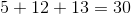inches.

3 feet are equivalent to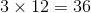inches, so this is the greater quantity.

### Example Question #1 : How To Find Length Of A Line

Which is the greater quantity?

(a) 370 meters

(b) 3,700 centimeters

(a) is the greater quantity

(b) is the greater quantity

(a) and (b) are equal

It is impossible to determine which is greater from the information given

(a) is the greater quantity

Explanation:

One meter is equivalent to 100 centimeters, so 370 meters can be converted to centimeters by multiplying by 100: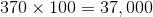.

370 meters are equal to 37.000 centimeters and is the greater quantity.

### Example Question #2 : How To Find Length Of A Line

Which is the greater quantity?

(a) 4.8 kilometers

(b) 4,800 meters

It is impossible to determine which is greater from the information given

(a) is the greater quantity

(a) and (b) are equal

(b) is the greater quantity

(a) and (b) are equal

Explanation:

One kilometer is equal to 1,000 meters, so convert 4.8 kilometers to meters by multiplying by 1,000: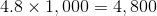meters

The two quantities are equal.

### Example Question #1 : How To Find Length Of A Line

A regular pentagon has perimeter 1 yard. Give the length of one side.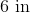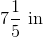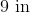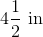Explanation:

A regular pentagon has five sides of equal length. The perimeter, which is the sum of the lengths of these sides, is one yard, which is equal to 36 inches. Therefore, the length of one side is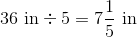.

### Example Question #3 : How To Find Length Of A Line

A regular hexagon has perimeter 8 feet. Give the length of one side.

16 inches

18 inches

24 inches

12 inches

16 inches

Explanation:

The perimeter can be converted from feet to inches by multiplying by conversion factor 12 {inches per foot):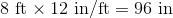A regular hexagon has six sides of equal length. Divide this perimeter by 6 to obtain the length of each side: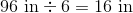### Example Question #1 : How To Find The Volume Of A Net

Which is the greater quantity?

(a) The sidelength of a cube with surface area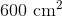(b) The sidelength of a cube with volume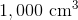It is impossible to tell from the information given

(a) and (b) are equal

(b) is greater

(a) is greater

(a) and (b) are equal

Explanation:

(a) A cube has six faces, each a square. Since the surface area of this cube is, each face has one-sixth this area, or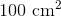; the sidelength is the square root of this, or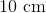.

(b) The volume of a cube is the cube of its sidelength, so we take the cube root of the volume of this cube to get the sidelength: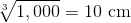The cubes have the same sidelength.

### Example Question #1 : How To Find The Volume Of A Net

Which is the greater quantity?

(a) The volume of a rectangular prism with length 60 centimeters, width 30 centimeters, and height 15 centimeters

(b) The volume of a cube with sidelength 300 millimeters

(a) and (b) are equal

(b) is greater

(a) is greater

It is impossible to tell from the information given

(a) and (b) are equal

Explanation:

(a) The volume of the prism is the product of its length, its width, and its height: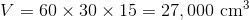(b) The volume of the cube is the cube of its sidelength. We restate 300 millimeters as 30 centimeters, and cube this: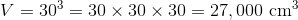The volumes are equal.

1 2 19 20 21 22 23 24 25 27 Next →

### All ISEE Middle Level Quantitative Resources